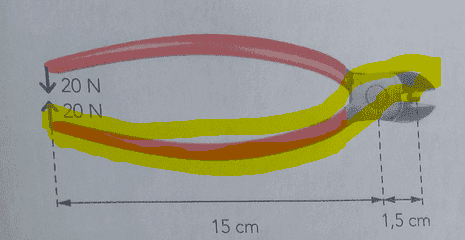# M=Fl solve a Moments problem (force X distance)

• Drizzy

#### Drizzy

1. The problem statement, all variables and given

http://imgur.com/kxALo8w

with how much force is the wire pinched?

M=Fl

## The Attempt at a Solution

[/B]
I don't know how I am supposed to do this. This is my attempt:

0,15 * 20 = 3Nm

and now what?

Last edited by a moderator:

and now what?
Use the same formula again. This time you know torque and distance and want to get the force.

but the force is 20 and 20 so I donät know what to do..

Forget the 20 N force, you used that to calculate torque, it is not necessary any more. Yes you have the force from both sides, but you also want to apply it on both sides at the wire, so that doesn't matter.

You have a torque of 3 Nm and you use it to apply a force at a distance of 1.5 cm. What is the force?

the force is 200N but I don't understand why that's how it is done

Because M=F*l works.

Alternatively, consider the lever arms. They have a length ratio of 10:1, so force has a ratio of 1:10. You can apply a force of 200 N on the wire from both sides.

hmm O am confused :/ what if i did it like this:

20* 0,015=F*0,15

This force is not 200N

hmm O am confused :/ what if i did it like this:

20* 0,015=F*0,15

This force is not 200N
It looks like 200N to me !

It looks like 200N to me !

it is 2N

it is 2N
I didn't look closely enough.

You switched things up compared to post #1.

I didn't look closely enough.

You switched things up compared to post #1.
yes that's the thing. why don't I get 200 N in my second attempt? I think the problem is is that I don't understand the scenario to begin with I just can't seem to grasp it :(...

You swapped the distances, they should be at the other side of the equal sign, respectively.The pliers have two major pieces plus the pin as a pivot (fulcrum).

Each handle has a jaw at the other end, all one piece.

I have one such handle & jaw piece highlighted.

The 20N force (upward) on that handle produces a counter-clockwise torque, 3Nm, on that piece. The object in the jaw must produce a clockwise torque of 3Nm to have rotational equilibrium.

The torque pair on the other piece (handle &jaw) is totally independent from this. However, if the forces on the second piece don't cancel those on the first piece, the whole apparatus (pliers) will accelerate.

#### Attachments

I get it now! thank you so much for your explanation :)